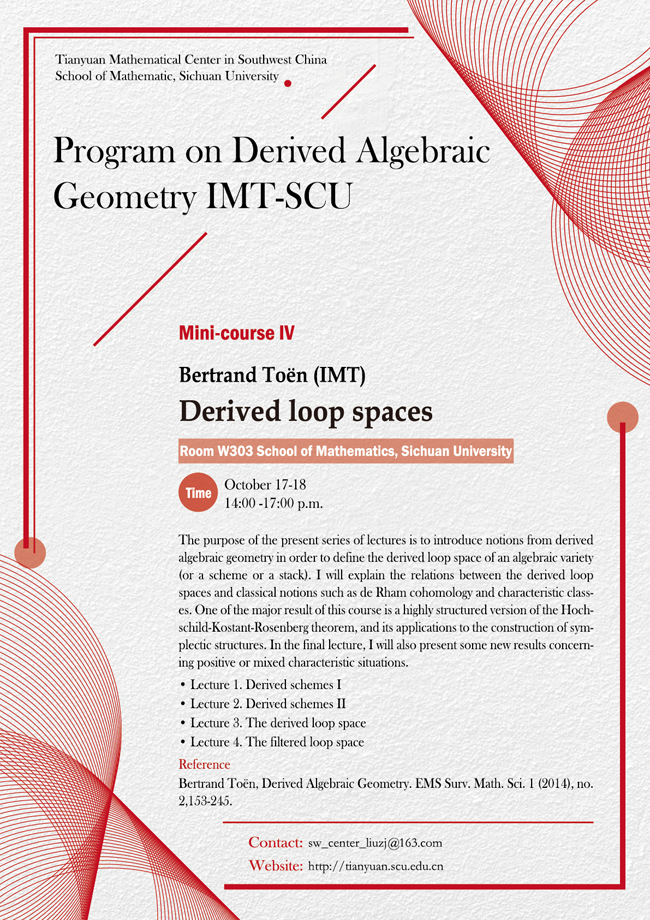2019 Thematic Program (IV)

### Derived loop spaces

#### Venue: W303  School of Mathematics, Sichuan University#### SPEAKER

Bertrand Toën (IMT)

#### ABSTRACT

The purpose of the present series of lectures is to introduce notions from derived algebraic geometry in order to define the derived loop space of an algebraic variety (or a scheme or a stack). I will explain the relations between the derived loop spaces and classical notions such as de Rham cohomology and characteristic classes. One of the major result of this course is a highly structured version of the Hochschild-Kostant-Rosenberg theorem, and its applications to the construction of symplectic structures. In the final lecture, I will also present some new results concerning positive or mixed characteristic situations.

• Lecture 1

Derived schemes I: simplicial commutative rings and their homotopy theory, examples.

• Lecture 2

Derived schemes II: derived schemes as ringed spaces, derived stacks as functors, cotangent complexes and formal comple- tion. Example : the derived moduli space of representations.

• Lecture 3

The derived loop space: definition and examples, the HKR theorem in characteristic zero, shifted symplectic structures.

• Lecture 4

The filtered loop space: the filtered circle and the HKR- filtration, applications to symplectic structures and the theory of sin- gular supports in non-zero characteristic.

#### reference

Bertrand Toën, Derived Algebraic Geometry. EMS Surv. Math. Sci. 1 (2014), no. 2, 153-245.